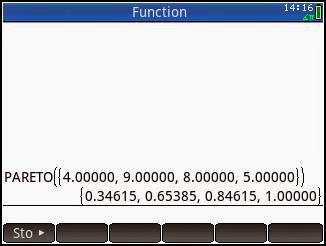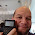## Saturday, July 26, 2014

### HP Prime: Drawing the Pareto Curve

This program draws the Pareto Curve given a list of frequencies.

Program Name:  PARETO(list of frequencies)

This is best called from the Home Screen and not the program catalog.  The curve drawn is not tracable, but is able to used without having to switch apps.

EXPORT PARETO(flist)
// 2014-07-25 EWS
BEGIN
// frequency list
LOCAL lx,ly,I,J,n;
// Setup
n:=SIZE(flist);
lx:=MAKELIST(X,X,1,n);
ly:=flist/ΣLIST(flist);
ly:=REVERSE(SORT(ly));
ly:=cumSum(ly);
// Draw the Curve
RECT();
Xmin:=0; Xmax:=n+1; Xtick:=1;
Ymin:=0; Ymax:=1.1; Ytick:=0.1;

FOR I FROM 1 TO n-1  DO
LINE(lx(I),ly(I),lx(I+1),ly(I+1),#FFh);
END;

FOR I FROM 1 TO n DO
TEXTOUT(ROUND(ly(I),2),lx(I),ly(I),1,#80h);
TEXTOUT(I,I,0,1,#FF0000h);
LINE(I,0,I,1,#D0D0D0h);
END;

FOR I FROM 0.1 TO 1 STEP 0.1 DO
LINE(0,I,n,I,#D0D0D0h);
END;

WAIT(0);
RETURN ly;
END;

Example:  A list of frequencies:  {4, 8, 9, 5}.  Note that the biggest frequency is plotted first, as the program first arranges the frequencies from largest to smallest.  Each point will show the cumulative percentage of the total population.  A list of the cumulative percentages will be returned to the home screen.Pareto Curve PlotList of Cumulative Percentages

Eddie

This blog is property of Edward Shore.  2014

#### 2 comments:

1.Hey There! Do you know any a function that goes through a list and returns if a value shows up multiple times, and how many repetirions of that value there are?
Trying to develop a Root Locus program for myselft, while studying the prime

Still no idea how to plot a graphic though.. :)
Pedro

1.Pedro,

No there are no built-in functions where it counts the number times an element is present in a list. This would require a program:

EXPORT COUNT(list,target)
BEGIN
// list then target
// n is the count
LOCAL n,i,s;
n:=0;
s:=SIZE(list);
FOR i FROM 1 TO s DO
IF list(i)==target THEN
n:=n+1;
END;
END;
RETURN n;
END;

Regarding a graphic, do you mean plot a function, parametric curve, geometric shapes, etc.?

Eddie

### Swiss Micros DM16L: Advanced Boolean and Factorial (up to 20)

Swiss Micros DM16L:   Advanced Boolean and Factorial (up to 20) Introduction The program listing, for the Swiss Micros DM16L and Hewlett Pac...